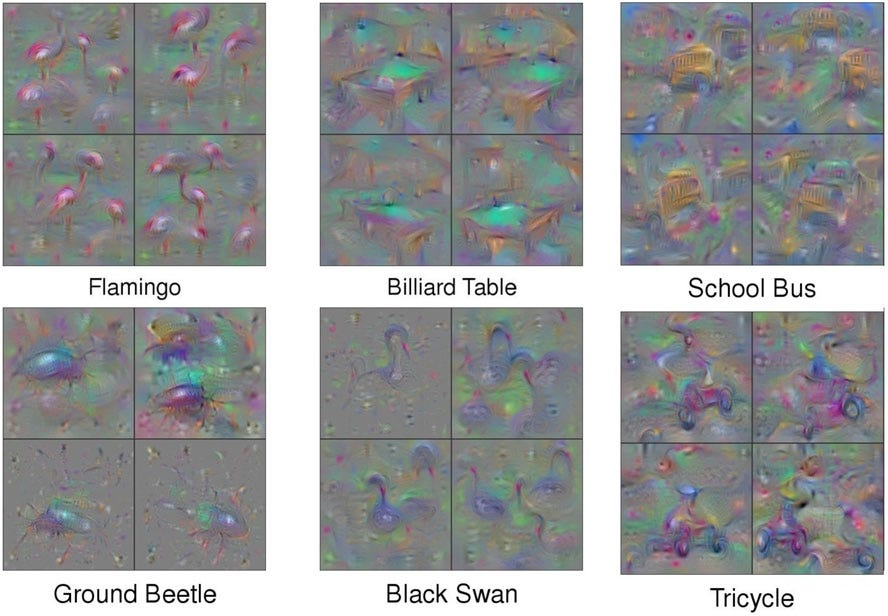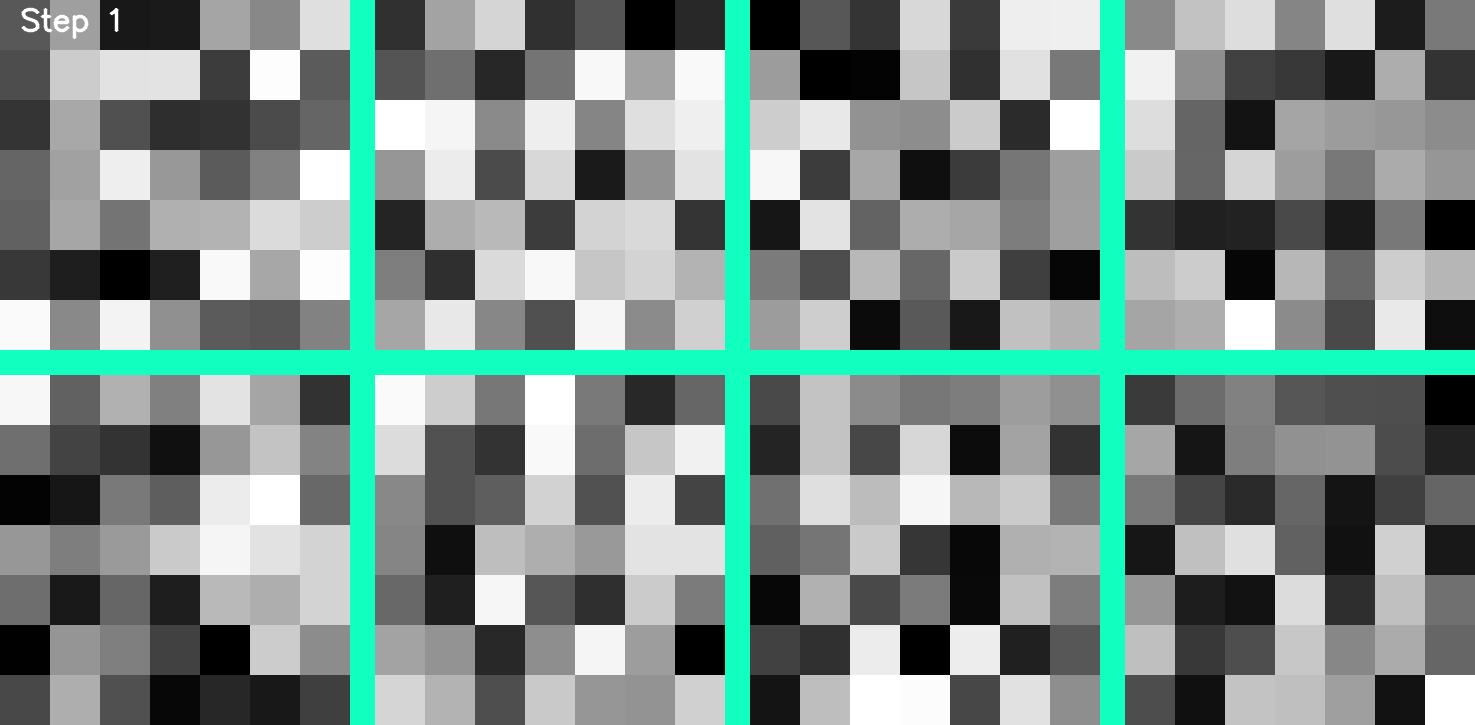# Convolution Neural Network Decryption

Source: Deep Learning on Medium

This article is an attempt to explain convolution neural networks via visualizations of very simple images. Very basic objects like vertical and horizontal lines are used. Complex images from ImageNet dataset have been avoided as the visualizations are not available for easy interpretations.

When I started with Deep Learning for a Computer Vision project a year back in 2017, the first tutorial I came across was the cat/dog classification and the MNIST example using Convolution Neural Network like many other people. Though these examples give us a broad understanding of how CNN works and its incredible efficiency it is difficult to really understand the inner workings. I saw that, there are a number of articles on building CNNs from scratch using different frameworks and also on various data sets to build classifiers. There is no doubt that nowadays anyone can build a pretty good image classification model under 30 mins using any of the many famous frameworks.

I am not going to get into the math of gradient descent and back-propagation because there are enough resources already available. Many of us do understand the math but still feel that we are missing something. A lot of people feel the same way and have been trying to figure out ways to understand what the BLACK BOX really learns and how to tune them better, since the advent of Deep Neural Networks.

There are a lot of visualization techniques created by many people to understand what the CNNs learn, and I am sure many of you would have come across a lot of weird colorful images which would give us little idea or even worse, make us feel like we do not understand anything at all like the ones below:

I feel that the major reason for this is because all the examples that we experiment with are all high level images. The Cat/Dog, ImageNet, or even MNIST data set are too complex of a problem for someone who has no experience in Computer Vision.

So, here I am going to explain CNNs with a very simple dataset of Horizontal lines and Vertical Lines. I generated around 600 images of size 50×50 in each class as shown below, and we are going to classify them using CNN:

Now imagine that you were given a task to write an algorithm from scratch to classify the images. This would be a pretty simple task for anyone with some background in Computer Vision, because all we need to do is implement a basic edge detection algorithm. There are a handful of algorithms which have been used for edge detection for many years now.

I will be demonstrating the Prewitt operator here but feel free to try other algorithms too. Prewitt operator is used for edge detection in an image. It detects two types of edges:

• Horizontal edges
• Vertical Edges

Edges are calculated by using difference between corresponding pixel intensities of an image. Mathematically, the operator uses two 3×3 kernels which are convolved(see GIF to get a basic idea) with the original image to calculate approximations of the derivatives — one for horizontal changes, and other for the vertical.

where * here denotes the 1-dimensional convolution operation. Gx and Gy will find the vertical and horizontal edges respectively. It simply works like a first order derivative and calculates the difference of pixel intensities in a edge region. As the center column is zero it does not include the original values of an image but rather it calculates the difference of right and left(or top and bottom) pixel values around that edge. This increases the edge intensity and it becomes enhanced comparatively to the original image.

Now lets come back to our CNN where we have multiple layers of Convolutions, Maxpooling, ReLU, Dropout etc. Out of the many layers the Convolution Layer is one of the layers where there are trainable parameters, which are the kernels. Kernels are also more famously called as weights in the Data Science community.

I trained my dataset on a fairly simple CNN model as shown below:

I used a 7×7 kernel for convolving. From the above model, it can be seen that I used 8 kernels in the first layer and thus the 400 trainable parameters as calculated below:

I had saved the weights of all the layers in each epoch, and visualized the progress of the training. The kernel values range between 0 to 1, and the visualization was created using matplotlib’s color map, so the color mapping would not accurately reproduce the actual values but it would give us a good idea of the distribution of values. Below is a GIF of the kernels after each epoch of training.Kernel visualization during training

The 7×7 kernels are visualized below, when they were randomly initialized and then the same kernels after training over the data set.

Comparing the before and after training kernels we would not be able to very clearly understand what each kernel has learnt. This can be better understood by convolving the kernels on top of images and passing the images through the ReLU and Maxpooling layers. For easier understanding I have selected two of the kernels which were very well activated for a horizontal and vertical edge.

The Kernel 5 and 8 before training when convolved on input images have very vague outputs and no clear activation. But after training the Kernel 5 activates the Vertical line well and not the horizontal line. To be even more specific it searches for edges from left to right direction. If you carefully look at Kernel 5 you can see that the left side of the kernel is closer to 0 and the right side is closer to 1. A similar pattern can be seen in Kernel 8, where the horizontal line gets activated.

So, from the above images we can understand that by back propagation, the model has learned to come up with something like a Prewitt operator’s Kernel on its own from just understanding the dataset. Only difference here is that there are many kernels and each kernel learns something(except for a few dead kernels).

Now if we go to next layers the kernels starts to learn higher level features when compared to edge detection, such as low and high level pattern recognition. But the problem is, it is difficult to visualize the higher level features because of the volume of the trainable parameters present even in a small model. I am not including the visualizations here, because there will be a lot of data, and would be difficult to make sense. I will write about it in a different article.

Below I have visualized my model, on an input horizontal line image, from beginning to end just to give an idea of how an image progresses in the next layers:

I hope this article helped you understand CNNs better. Please share your feedbacks or doubts below.